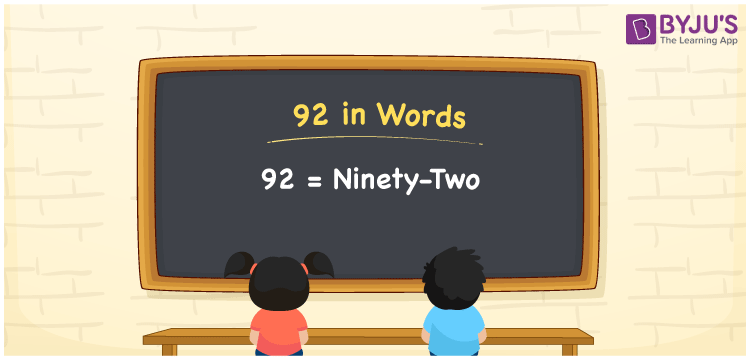# 92 in Words

92 in words is written as “Ninety-two”. In Mathematics, 92 is a natural number or an integer that is used to represent a value or a quantity. If you give 92 rupees to your friend, then you can say “I have given Rupees Ninety-two to him”. Hence, 92 in words is used not only in Maths but in real life too. Learn more about Numbers In Words at BYJU’S.

 92 in Words Ninety-two Ninety-two in Numbers 92

## 92 in English Words

92 in Words using English alphabets is expressed as given below:## How to Write 92 in Words?

We will use here place value method to write 92 in words. The below place value chart for number 92, represents the position of each digit.

 Tens Ones 8 2

From the above table, we can see, 9 is at tens place and 2 is at ones place. We need to read the number from right to left, in the table. Thus, together we can write the number as Ninety-two.

### Expanded Form of 92

We can write the expanded form of 92 as:

9 at Tens + 2 at Ones

= 9 × 10 + 2 × 1

= 92

= Ninety-two

92 is a whole number that is succeeded by 91 and preceded by 93. Find more about the number 92 below:

• 92 in Words – Ninety-two
• Is 92 an odd number? – No
• Is 92 an even number? – Yes
• Is 92 a perfect square number? – No
• Is 92 a perfect cube number? – No
• Is 92 a prime number? – No
• Is 92 a composite number? – Yes

## Frequently Asked Questions on 92 in words

### What is 92 in words?

92 in words is given by Ninety-two.

### What is the rule to write 92 in words?

92 in words is written based on the place values of each digit. In 92, 9 is at tens place and 2 is at units place. Thus, ninety plus two is read as ninety-two.

### How to spell 92 in English words?

92 in English is spelt as Ninety-two.